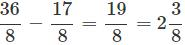# Test: Fraction - 2

## 10 Questions MCQ Test Mathematics (Maths) for Class 4 | Test: Fraction - 2

Description
This mock test of Test: Fraction - 2 for Class 4 helps you for every Class 4 entrance exam. This contains 10 Multiple Choice Questions for Class 4 Test: Fraction - 2 (mcq) to study with solutions a complete question bank. The solved questions answers in this Test: Fraction - 2 quiz give you a good mix of easy questions and tough questions. Class 4 students definitely take this Test: Fraction - 2 exercise for a better result in the exam. You can find other Test: Fraction - 2 extra questions, long questions & short questions for Class 4 on EduRev as well by searching above.
QUESTION: 1

### 3 times of 25/81 = ______.

Solution:

3 times of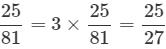QUESTION: 2

### What fraction of the figure is unshaded?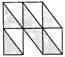Solution:

Total number of equal parts =10 Number of unshaded parts = 4
∴ Required fraction = 4/10

QUESTION: 3

### What fraction of the figure is unshaded?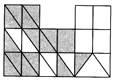Solution:

Total number of equal parts = 32 Number of unshaded parts =16
∴ Required fraction = 16/32 = 1/2 i.e., one half

QUESTION: 4

What fraction of the figure is shaded?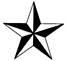Solution:

Total number of equal parts =10 Number of shaded parts = 5
∴ Required fraction = 5/10

QUESTION: 5

The model is shaded to show which fraction?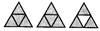Solution:

The model shows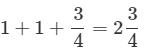QUESTION: 6

Which of the following shaded fraction represent less than the shaded fraction of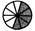?

Solution:
QUESTION: 7

DIRECTION: Yashika has a bag with 8 red marbles, 4 blue marbles, 5 green marbles and 9 yellow marbles all of the same size.
What fraction of marbles are red?

Solution:

Total number of marbles in the bag =8+4+5+9=26 Number of red marbles = 8
∴ Required fraction = 8/26

QUESTION: 8

DIRECTION: Yashika has a bag with 8 red marbles, 4 blue marbles, 5 green marbles and 9 yellow marbles all of the same size. What fraction of marbles are not yellow?

Solution:

Number of marbles which are not yellow =17
∴ Required fraction = 17/26

QUESTION: 9

What fraction of the figure is shaded?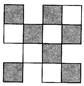Solution:

Total number of equal parts =12 Number of shaded parts = 7
∴ Required fraction = 7/12

QUESTION: 10

The difference between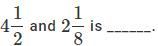Solution: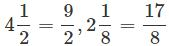Converting the fractions into like fractions LCM of 2 and 8 = 8
∴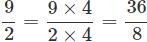and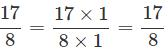Now,